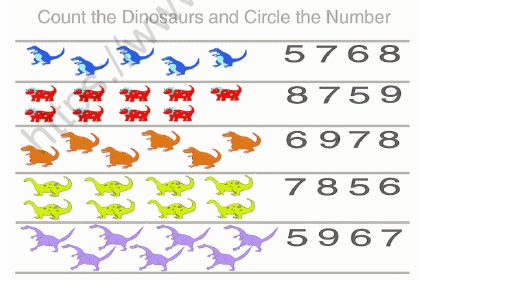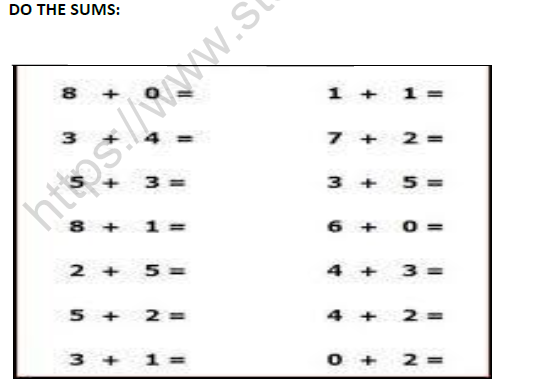# CBSE Class 1 Maths Addition Worksheet Set E

Download printable Mathematics Class 1 Worksheets in pdf format, CBSE Class 1 Maths Addition Worksheet Set E has been prepared as per the latest syllabus and exam pattern issued by CBSE, NCERT and KVS. Also download free pdf Mathematics Class 1 Assignments and practice them daily to get better marks in tests and exams for Grade 1. Free chapter wise worksheets with answers have been designed by Standard 1 teachers as per latest examination pattern

## Worksheet for Class 1 Mathematics Addition

Class 1 Mathematics students should refer to the following printable worksheet in Pdf for Addition in Grade 1. This test paper with questions and solutions for Standard 1 Mathematics will be very useful for tests and exams and help you to score better marks

### Addition Class 1 Mathematics Worksheet Pdf

Circle the numbers you add first. Write the sum.

1. 8 + 6 + 4 = _________

2. 6 + 6 + 3 = _________

3. 9 + 8 + 2 = _________

4. 4 + 6 + 5 = _________

5. 7 + 8 + 2 = _________

Write the sum.Subtract Tens

1.  What is 2 tens from 3 tens?

2.  What is 5 tens from 9 tens?

3.  What is 1 ten from 3 tens?

4.  What is 4 tens from 8 tens?